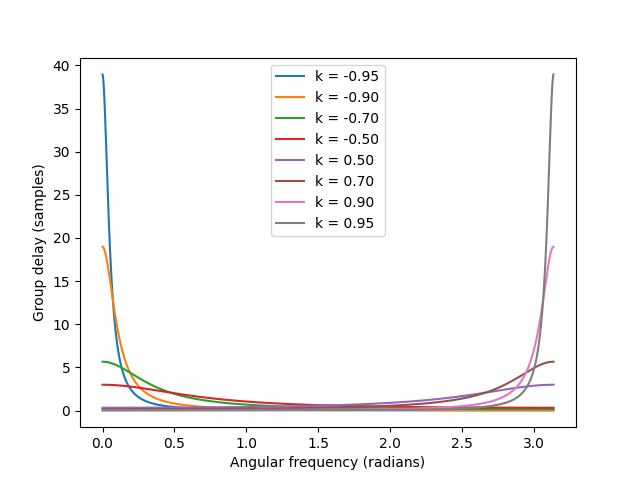# LSP parametric equalizer. What does the allpass filter do?

The LSP parametric equalizer has an allpass filter. But, what do the controls do in this regard? How does the phase change?

Related to supercollider because i’m using it to process some tracks.

Depends on the type of allpass. In this case it’s probably a 2nd-order.

Generally a low-order allpass is not very audible unless it’s in a feedback loop or you cascade many copies of it. (Although I do know a mastering engineer who claims he can hear the phase distortion of a low-order IIR lowpass or highpass.)

Yeah it doesn’t say how much of a delay it adds, or whether it works in a certain bandwidth or if it’s a fullband allpass. On the gui, the dot handle in the spectroscope moves along the frequency range, but not the gain or the Q. There isn’t much other documentation either.

If there’s no Q control then it may just be a first-order allpass. Transfer function of such a filter is `H(z) = (k + z^-1)/(1 + kz^-1)`. This Python script graphs the group delay for a few choice values of k. The Y-axis is the frequency-dependent delay in samples, the X-axis is the angular frequency in radians (0 is DC, pi is Nyquist).

``````import numpy as np
import matplotlib.pyplot as plt

for k in [-0.95, -0.9, -0.7, -0.5, 0.5, 0.7, 0.9, 0.95]:
w = np.linspace(0, np.pi, 1024)
z = np.exp(w * 1j)
z1 = 1 / z
H = (k + z1) / (1 + k * z1)
phase = np.angle(H)
group_delay = -np.diff(np.unwrap(phase)) / np.diff(w)
plt.plot(w[:-1], group_delay, label=f"k = {k:.2f}")

plt.ylabel("Group delay (samples)")
plt.legend()
plt.show()

``````edit: mistake in axis labels fixed

So the graph indicates how many samples get delayed at which frequency? And k is a scaling term?
What would a Q control do?

Yes. However, group delay doesn’t give you the whole picture of an allpass filter. A filter with a constant nonzero phase rotation has zero group delay at all frequencies, but still produces a different signal. The Hilbert transform is one such filter. So is a simple polarity inversion, which rotates all phases by 180 degrees. (The group delay is -1 times the derivative of the phase response with respect to angular frequency. Recovering the phase response from the group delay requires figuring out the + C in your indefinite integral.)

Also, while frequency-dependent delays are an important side effect of filter designs, low-order IIR allpass filters are not implemented using traditional ring-buffer-style delay lines like DelayN.

No, it’s a filter parameter that will give you different phase responses.

I’m fairly certain Q isn’t a meaningful concept for a first-order allpass filter. It is however meaningful for second-order allpass filters, so if an allpass filter has a Q control it’s probably second-order. It’s late here, so I can’t code up a graph right now, but IIRC the group delay is bell-shaped. There’s a band of frequencies where the group delay reaches a maximum, and it smoothly tapers off to both sides.